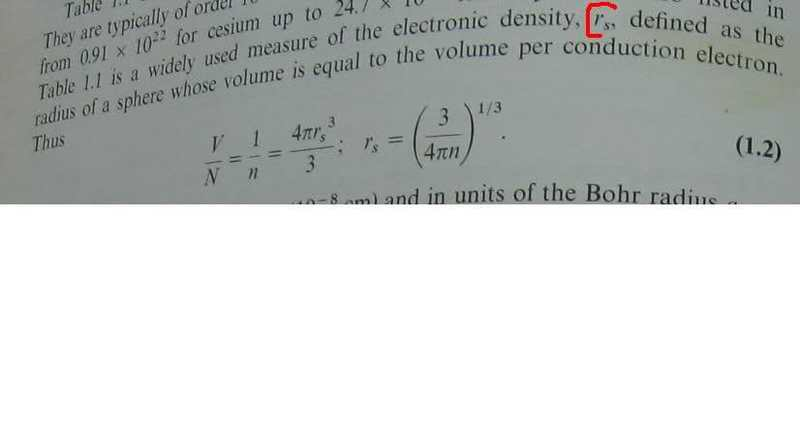# Calculating Sphere Volume with Conductive Electrons

• nhrock3

#### nhrock3r_s is the radius of a sphere volume equals ...
what is volume per conduction electron?

what is this volume like?

what is this volume like?

Volume is like another other volume, it's a 3D region of space. In this case the region is taken to be sphere of radius r_n. And within that volume you have N number of conduction electrons.

Divide that and you get a density of conduction electrons per volume or vice-versa.

"Divide that and you get a density of conduction electrons per volume or vice-versa."

divide what?and by what?
density is a mass divided by volume
there is no such thing here

Density, in this case, refers to the number (not the mass) per unit volume. It (or the reciprocal) tells you how much room a conduction electron has 'to itself' or the space between conduction electrons.
When we talk of Population Density, we don't mean the Mass, either. It's a fairly common English / Technical use of the term density.